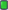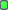# maths test

Forum RegularThread Starter

Joined: May 2013
Posts: 126maths test

This may sound like a silly question but does anyone know if we are allowed to use a calculator in the maths test? I am fairly good at maths and I know the theories behind percentage finding etc but when it comes to long division and multiplication in this day and age do we need to work it out ourselves? For example if a question was in a school of 850 students 319 were females so what is this percentage. Its easy to know it would be 319÷850 × 100 would give the answer but could we use a calc for the sum part?Forum RegularJoined: Jun 2013
Location: London
Posts: 80Re: maths test

This may sound like a silly question but does anyone know if we are allowed to use a calculator in the maths test? I am fairly good at maths and I know the theories behind percentage finding etc but when it comes to long division and multiplication in this day and age do we need to work it out ourselves? For example if a question was in a school of 850 students 319 were females so what is this percentage. Its easy to know it would be 319÷850 × 100 would give the answer but could we use a calc for the sum part?
No calculators allowed. SorryJoined on April fools dayJoined: Apr 2012
Location: Hops capital of...well hops festival
Posts: 10,638Re: maths test

This may sound like a silly question but does anyone know if we are allowed to use a calculator in the maths test? I am fairly good at maths and I know the theories behind percentage finding etc but when it comes to long division and multiplication in this day and age do we need to work it out ourselves? For example if a question was in a school of 850 students 319 were females so what is this percentage. Its easy to know it would be 319÷850 × 100 would give the answer but could we use a calc for the sum part?
Doing it without the calculator helps you to understand basic math more completely. Rather than just looking at the answer.Forum RegularThread Starter

Joined: May 2013
Posts: 126Re: maths test

Fair enough but it seems quite silly. Surley by getting to the correct answer you are showing that you understand maths. In this day and age who would need to work out long multiplication etc? Even if you were the best mathematician in the world if you have a mobile phone or a calc why would u waste time doing long sums? Oh well ill have to get my gcse bite size books from the loftChillin out in WAJoined: Jul 2003
Location: Mandurah, WA
Posts: 259Re: maths test

Don't look at it as a maths test.
It's a mental capacity test, to see if your mental capacity can deal with complex problems.You may not post new threads
You may not post replies
You may not post attachments
You may not edit your posts

BB code is On
Smilies are On
[IMG] code is On
HTML code is Off
Trackbacks are Off
Pingbacks are Off
Refbacks are Off

Thread ToolsShow Printable VersionEmail this Page

Copyright © 2018 MH Sub I, LLC dba Internet Brands. All rights reserved. Use of this site indicates your consent to the Terms of Use.